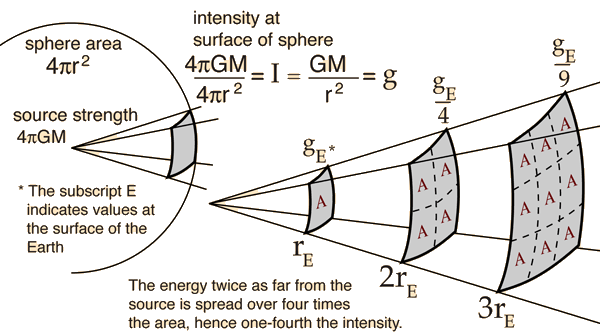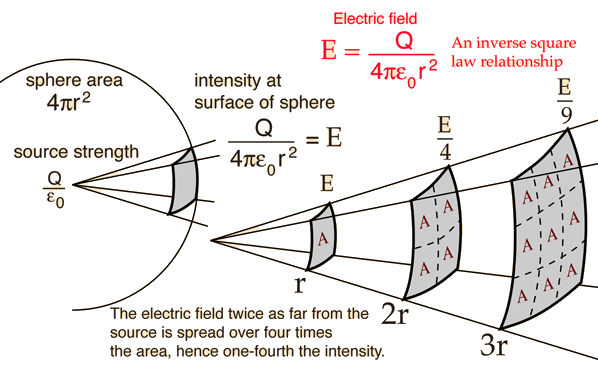# Inverse Square Law, General

Any point source which spreads its influence equally in all directions without a limit to its range will obey the inverse square law. This comes from strictly geometrical considerations. The intensity of the influence at any given radius r is the source strength divided by the area of the sphere. Being strictly geometric in its origin, the inverse square law applies to diverse phenomena. Point sources of gravitational force, electric field, light, sound or radiation obey the inverse square law. It is a subject of continuing debate with a source such as a skunk on top of a flag pole; will its smell drop off according to the inverse square law?Applications of the inverse square law:
 Gravity Electric field Light Sound Radiation
Index

 HyperPhysics***** Mechanics R Nave
Go Back

# Inverse Square Law, Gravity

As one of the fields which obey the general inverse square law, the gravity field can be put in the form shown below, showing that the acceleration of gravity, g, is an expression of the intensity of the gravity field.Other applications of the inverse square law Electric field Light Sound Radiation
Index

Gravity concepts

 HyperPhysics***** Mechanics R Nave
Go Back

# Inverse Square Law, Electric

As one of the fields which obey the general inverse square law, the electric field of a point charge can be put in the form shown below where point charge Q is the source of the field. The electric force in Coulomb's law follows the inverse square law.Other applications of the inverse square law Gravity Light Sound Radiation
Index

Gravity concepts

 HyperPhysics***** Electricity and Magnetism R Nave
Go Back

# Inverse Square Law, Radiation

As one of the fields which obey the general inverse square law, a point radiation source can be characterized by the relationship below whether you are talking about Roentgens , rads, or rems . All measures of exposure will drop off by inverse square law.The source is described by a general "source strength" S because there are many ways to characterize a radiation source - by grams of a radioactive isotope, source strength in Curies, etc. For any such description of the source, if you have determined the amount of radiation per unit area reaching 1 meter, then it will be one fourth as much at 2 meters.

 Other applications of the inverse square law Gravity Light Sound Electric
Index

Gravity concepts

 HyperPhysics***** Radioactivity R Nave
Go Back